Question

A particle that carries a net charge of -95.8 μC is held in a region of constant, uniform electric field. The electric field vector is oriented 70.2° clockwise from the vertical axis, as shown. If the magnitude of the electric field is 5.32 N/C, how much work is done by the electric field as the particle is made to move a distance of d = 0.956 m straight up?

What is the potential difference between the particle\'s initial and final positions (Vf - Vi)?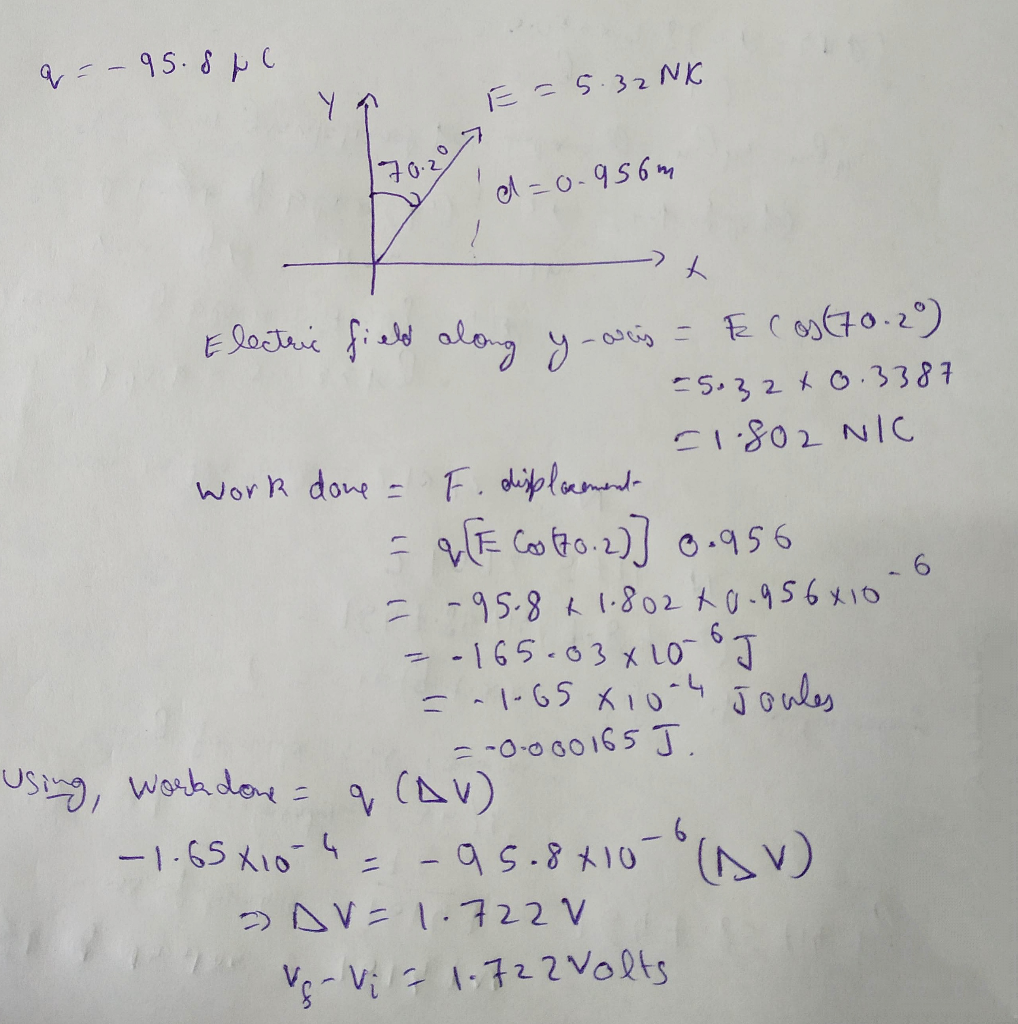#### Earn Coins

Coins can be redeemed for fabulous gifts.

Similar Homework Help Questions
• ### A particle that carries a net charge of -41.8 μC is held in a region of...

A particle that carries a net charge of -41.8 μC is held in a region of constant, uniform electric field. The electric field vector is oriented 55.2° clockwise from the vertical axis, as shown. If the magnitude of the electric field is 5.82 N/C, how much work is done by the electric field as the particle is made to move a distance of d = 0.156 m straight up? What is the potential difference between the particle\'s initial and final...

• ### A particle that carries a net charge of-59.8 μC is held in a region of constant,...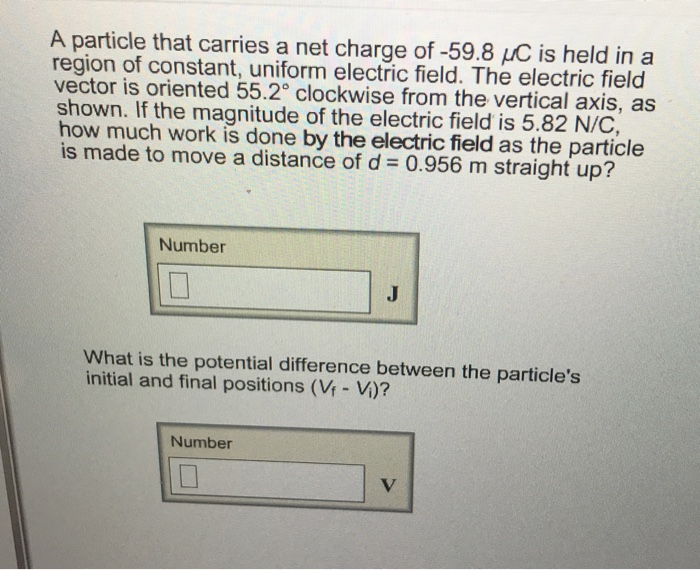A particle that carries a net charge of-59.8 μC is held in a region of constant, uniform electric field. The electric field vector is oriented 55.2° clockwise from the vertical axis, as shown. If the magnitude of the electric field is 5.82 N/C, how much work is done by the electric field as the particle is made to move a distance of d = 0.956 m straight up? Number What is the potential difference between the particle's initial and final...

• ### A particle that carries a net charge of -41.8 HC is held in a region of...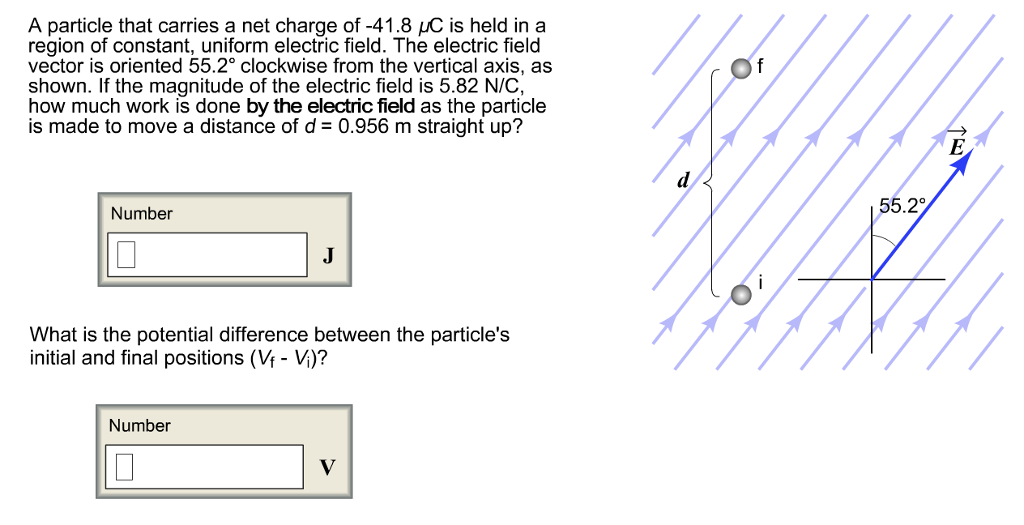A particle that carries a net charge of -41.8 HC is held in a region of constant, uniform electric field. The electric field vector is oriented 55.2° clockwise from the vertical axis, as shown. If the magnitude of the electric field is 5.82 N/C how much work is done by the electric field as the particle is made to move a distance of d- 0.956 m straight up? 55.20 Number What is the potential difference between the particle's initial and...

• ### particle that carries a net charge of-41.8 LC is held in a region of constant, uniform...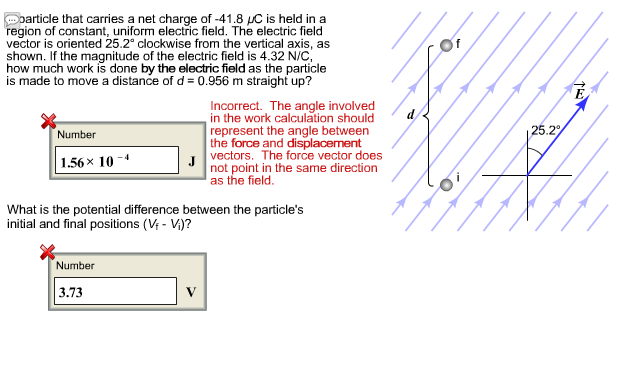particle that carries a net charge of-41.8 LC is held in a region of constant, uniform electric field. The electric field vector is oriented 25.2° clockwise from the vertical axis, as shown. If the magnitude of the electric field is 4.32 N/C how much work is done by the electric field as the particle is made to move a distance of d 0.956 m straight up? Incorrect. The angle involved in the work calculation should represent the angle between the...

• ### A particle that carries a net charge of -23.8 ?C A particle that carries a net...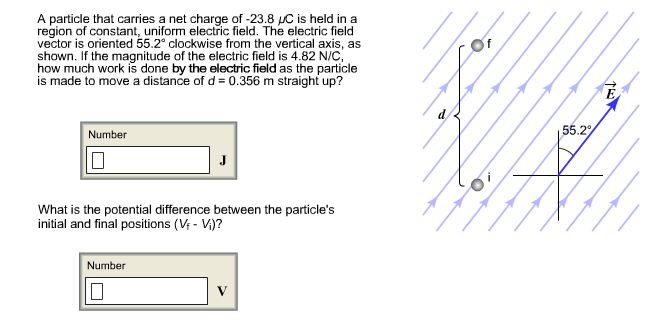A particle that carries a net charge of -23.8 ?C A particle that carries a net charge of -23.8 muC is held in a region of constant, uniform electric field. The electric field vector is oriented 55.2degree clockwise from the vertical axis, as shown. If the magnitude of the electric field is 4.82 N/C, how much work is done by the electric field as the particle is made to move a distance of d = 0.356 m straight up? What...

• ### 19 Questions Assignment Score: 100/1900 Resources Hint Check Answers Check Answer 1 Question Tocottempts 100/100 Correct...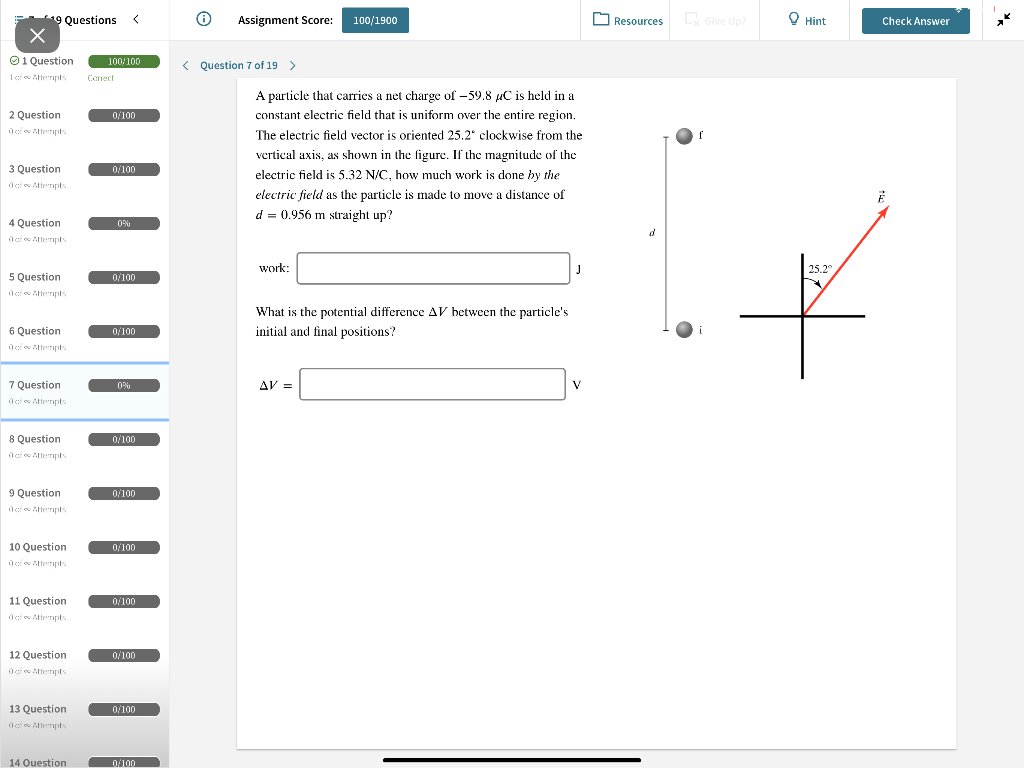19 Questions Assignment Score: 100/1900 Resources Hint Check Answers Check Answer 1 Question Tocottempts 100/100 Correct < Question 7 of 19 > 2 Question 0/100 A particle that carries a net charge of - 59.8 4C is held in a constant electric field that is uniform over the entire region. The electric field vector is oriented 25.2 clockwise from the vertical axis, as shown in the figure. If the magnitude of the electric field is 5.32 N/C, how much work...

• ### ty-PHYS 202 - Fall 19 - STINNETT > Activities and Due Dates > HW: Electric Potential...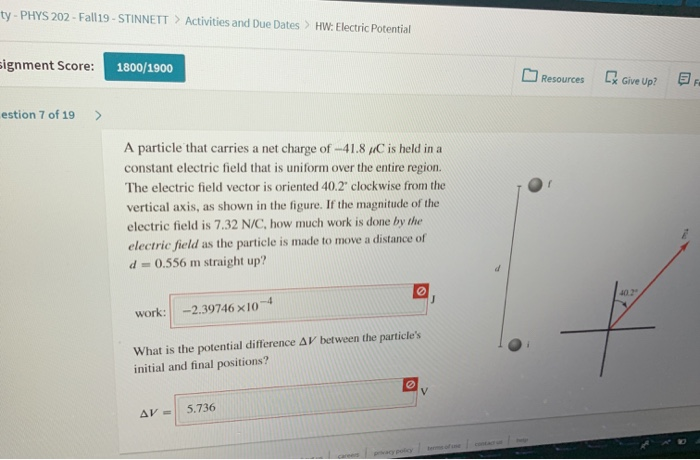ty-PHYS 202 - Fall 19 - STINNETT > Activities and Due Dates > HW: Electric Potential signment Score: 1800/1900 Resources De Give Up? BR estion 7 of 19 > A particle that carries a net charge of -41.8/C is held in a constant electric field that is uniform over the entire region. The electric field vector is oriented 40.2 clockwise from the vertical axis, as shown in the figure. If the magnitude of the electric field is 7.32 N/C, how...

• ### A charge of 20.0-μC is held fixed at the origin. A -5.0-μC charge with a mass...

A charge of 20.0-μC is held fixed at the origin. A -5.0-μC charge with a mass of 10.0 g is launched (outwards) from the position (1.0 m, 1.0 m) at a speed of 12.0 m/s. (a) At what distance from the origin will its speed be reduced to 6.0 m/s? (b) Will it ever halt? If yes, at what distance will it halt? Three point charges are located at the corners of an equilateral triangle, whose side l = 0.5...

• ### There are only two charged particles in a particular region. Particle 1 carries a charge of +q. Particle 2 carries a cha...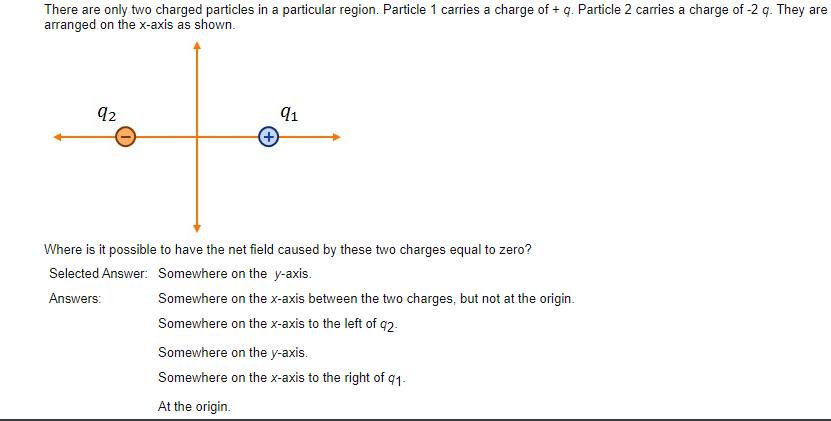There are only two charged particles in a particular region. Particle 1 carries a charge of +q. Particle 2 carries a charge of -2q. They are arranged on the x-axis as shown. Where is is possible to have the net field caused by these two charges equal to zero? Im thinking its at the origin maybe.... There are only two charged particles in a particular region. Particle 1 carries a charge of+ q. Particle 2 carries a charge of -2...

• ### The work done by an external force to move a particle of charge -2.20 μC at...

The work done by an external force to move a particle of charge -2.20 μC at constant speed from point A to point B is 6.40x10−310−3 J. (a) What is the difference between the electric potential energy of the charge at the two points? (b) What is the difference in the kinetic energy of the charge at the two points? (c) Determine the potential difference between the two points. (d) State which point is at the higher potential.